Exercises

# Multiplication 3

Multiplication is essentially repeated addition, or growing numbers by doubling, tripling, quadrupling, and so on. This unit presents mental models and strategies that help students learn and review the concept of multiplication. Students explore multiplication using arrays, partial products, doubling methods and the standard algorithm to solve numerical and word problems. Students will multiply whole numbers and simple decimals by base ten exponents (i.e. .3 x 103).
This lesson includes 5 printable learning activities.

## Double-Digit Array Multiplication with Radars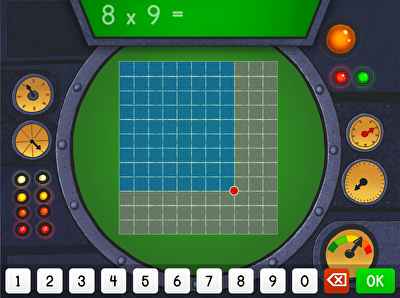Mayday, mayday! Muggo's fleet is in trouble. Fifth graders must calculate the product of smaller arrays to help Muggo's ship get back on course. Great for visual learners, this array game shows students how to determine the product of two-digit multiplication problems by dividing the area of a grid into smaller arrays. Practicing these skills help kids move from concrete to abstract problem-solving in multiplication.

## Multi-Digit Multiplication and Partial Products 2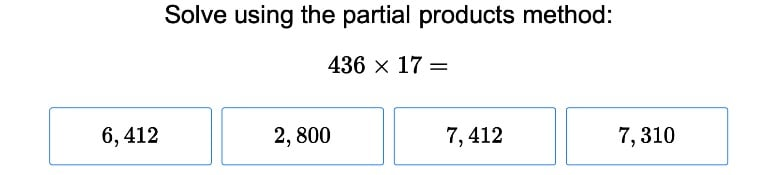For students just learning how to deal with partial products, this exercise will make the job less complicated.

## Multi-Digit Multiplication and Doubling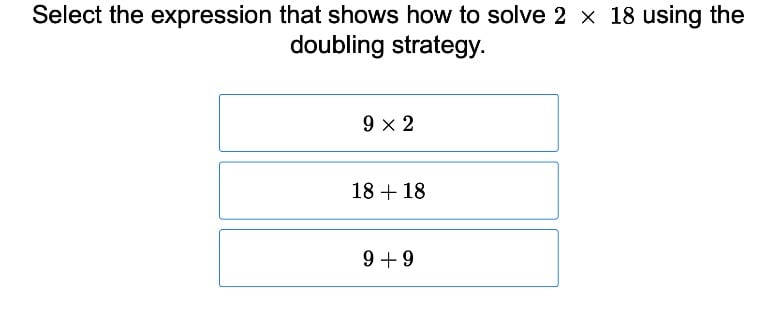Aid your fourth grader in learning multi-digit multiplication with these exercises that show students how to visualize doubling.

## Multi-Digit Multiplication 2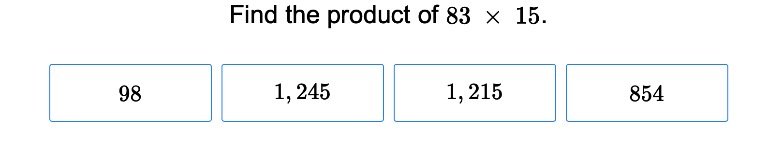Fifth graders are ready to learn how to multiply multi-digit numbers once they've mastered simpler problems and are looking for a challenge. Give students the practice they need to succeed with these exercises.

## Build a Pool: Area Models and Multiplication Word Problems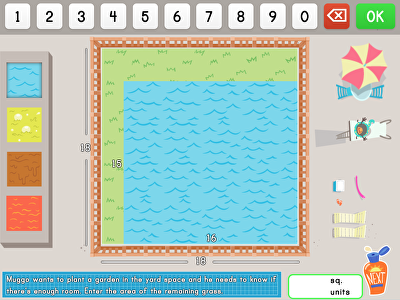Architects on the ready! Practice finding the area while giving kids the opportunity to build a pool in this hands-on math game. Kids will love having control of the game and letting their imaginations run wild while creating a pool with a set area. Follow-up word problems help kids take their backyard design a step further while using multiplication to answer questions about area.

## Multi-Digit Multiplication and Word Problems 1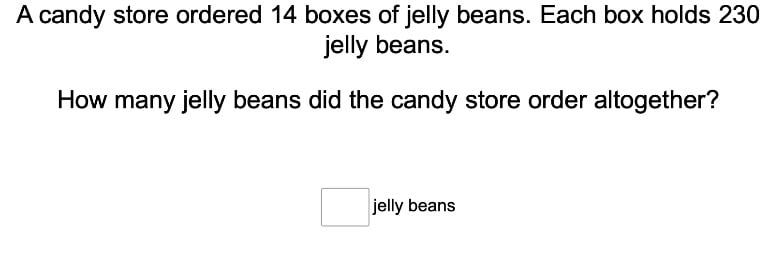Fourth graders apply their multiplication skills with these exercises that tie in school lessons with the real world in word problem form.

## Multiplying Decimal Numbers in Science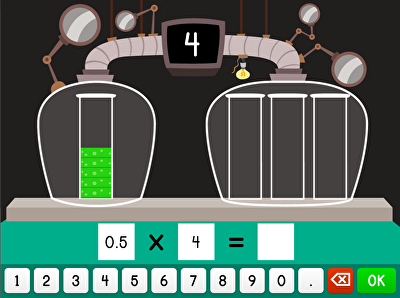Every good scientist needs to learn how to work with decimals. Your fifth grader can level up from regular multiplication to multiplying decimal numbers in this challenging game that helps Penelope with a science experiment. Each time your child completes an equation correctly, Penelope's beakers will fill to the amount in the answer, providing a handy way to visualize decimal numbers.

## Multiplying Decimals to the Tenths PlaceTeach your fifth grader the importance of multiplying by decimals with these exercises that give them actual situations and helpful hints.

## Multiplying Decimals to the Hundredths Place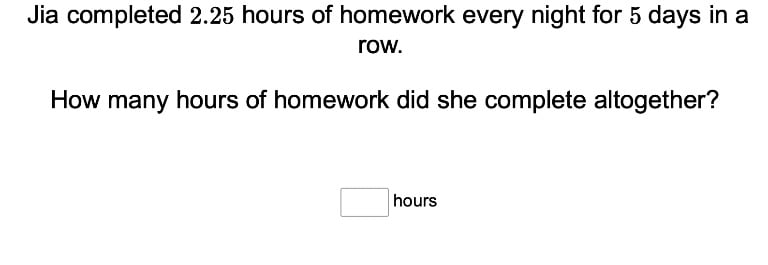Build on your students' knowledge of decimals and multiplication with these exercises that incorporate both skills together.

## Exponents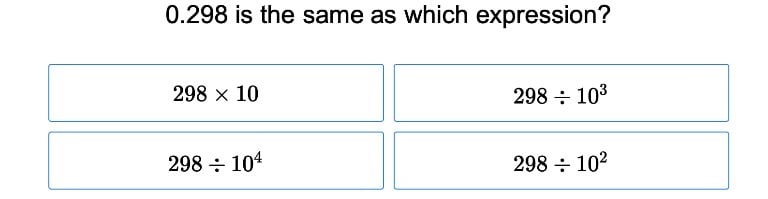Demystify the concept of exponents for students with a simple round of practice with this exercise.

## Multiplication and Powers of 10Have your students merge two skill sets with these exercises that incorporate powers of ten into multiplication problems.